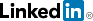cancel
Showing results for
Did you mean:
Highlighted
swapnil_singhalFrequent Visitor

## Minimum value of each group in a hierarchy in matrix visual

I've a dataset which looks as mentioned below:

 Level_1 Level_2 Value A A_1 1 A A_1 2 A A_2 3 A A_2 4 A A_2 5 B B_1 10 B B_1 11 B B_1 12 B B_1 13 B B_2 14 B B_2 15 B B_3 16 B B_3 17 B B_3 18

I want use the matrix visualization to aggregate the data and select the sum of minimum value at at each hierarchy level. Refer to screen shots below:The matrix visual shows the minimum value which is at next level of hierarchy, but I need the outcome at "15" which is minimum out of 15 and 126. Similarly if we go a level down:I need the output as 3 instead.

Any help will be very much appreciated.

1 ACCEPTED SOLUTION

Accepted Solutions
MariuszSenior Member

## Re: Minimum value of each group in a hierarchy in matrix visual

You can try the below DAX expression

```Min of Values =
VAR levelOne =
MINX(
ALL( YourTable[Level_1] ),
CALCULATE( SUM( YourTable[Value] ) )
)
VAR levelTwo =
MINX(
ALL( YourTable[Level_2] ),
CALCULATE( SUM( YourTable[Value] ) )
)
RETURN
SWITCH(
TRUE,
HASONEVALUE( YourTable[Level_2] ), levelTwo,
HASONEVALUE( YourTable[Level_1] ), levelOne,
SUM( YourTable[Value] )
)```

Best Regards,
Mariusz

If this post helps, then please consider Accepting it as the solution.

Please feel free to connect with me.2 REPLIES 2
MariuszSenior Member

## Re: Minimum value of each group in a hierarchy in matrix visual

You can try the below DAX expression

```Min of Values =
VAR levelOne =
MINX(
ALL( YourTable[Level_1] ),
CALCULATE( SUM( YourTable[Value] ) )
)
VAR levelTwo =
MINX(
ALL( YourTable[Level_2] ),
CALCULATE( SUM( YourTable[Value] ) )
)
RETURN
SWITCH(
TRUE,
HASONEVALUE( YourTable[Level_2] ), levelTwo,
HASONEVALUE( YourTable[Level_1] ), levelOne,
SUM( YourTable[Value] )
)```

Best Regards,
Mariusz

If this post helps, then please consider Accepting it as the solution.

Please feel free to connect with me.swapnil_singhalFrequent Visitor

## Re: Minimum value of each group in a hierarchy in matrix visual

Thanks a lot @Mariusz , solution worked perfectly fine.